# 5.6: Exercises

$$\newcommand{\vecs}{\overset { \rightharpoonup} {\mathbf{#1}} }$$ $$\newcommand{\vecd}{\overset{-\!-\!\rightharpoonup}{\vphantom{a}\smash {#1}}}$$$$\newcommand{\id}{\mathrm{id}}$$ $$\newcommand{\Span}{\mathrm{span}}$$ $$\newcommand{\kernel}{\mathrm{null}\,}$$ $$\newcommand{\range}{\mathrm{range}\,}$$ $$\newcommand{\RealPart}{\mathrm{Re}}$$ $$\newcommand{\ImaginaryPart}{\mathrm{Im}}$$ $$\newcommand{\Argument}{\mathrm{Arg}}$$ $$\newcommand{\norm}{\| #1 \|}$$ $$\newcommand{\inner}{\langle #1, #2 \rangle}$$ $$\newcommand{\Span}{\mathrm{span}}$$ $$\newcommand{\id}{\mathrm{id}}$$ $$\newcommand{\Span}{\mathrm{span}}$$ $$\newcommand{\kernel}{\mathrm{null}\,}$$ $$\newcommand{\range}{\mathrm{range}\,}$$ $$\newcommand{\RealPart}{\mathrm{Re}}$$ $$\newcommand{\ImaginaryPart}{\mathrm{Im}}$$ $$\newcommand{\Argument}{\mathrm{Arg}}$$ $$\newcommand{\norm}{\| #1 \|}$$ $$\newcommand{\inner}{\langle #1, #2 \rangle}$$ $$\newcommand{\Span}{\mathrm{span}}$$$$\newcommand{\AA}{\unicode[.8,0]{x212B}}$$

## Analysis

1. Given the circuit in Figure 7.6.1 , write the mesh loop equations.Figure 7.6.1

2. Using mesh analysis, determine the value of $$V_b$$ for the circuit shown in Figure 7.6.1 .

3. For the circuit shown in Figure 7.6.1 , use mesh analysis to determine the current through the 1 k$$\Omega$$ resistor.

4. Given the circuit in Figure 7.6.2 , write the mesh loop equations and the associated determinants.Figure 7.6.2

5. Using mesh analysis, determine the value of $$V_b$$ for the circuit shown in Figure 7.6.2 .

6. For the circuit shown in Figure 7.6.2 , use mesh analysis to determine the current through the 500 $$\Omega$$ resistor.

7. Given the circuit in Figure 7.6.3 , write the mesh loop equations.Figure 7.6.3

8. Using mesh analysis, determine the value of $$V_b$$ for the circuit shown in Figure 7.6.3 .

9. For the circuit shown in Figure 7.6.3 , use mesh analysis to determine the current through the 75 $$\Omega$$ resistor.

10. Given the circuit in Figure 7.6.4 , write the mesh loop equations and the associated determinants.Figure 7.6.4

11. Using mesh analysis, determine the value of $$V_{bc}$$ for the circuit shown in Figure 7.6.4 .

12. For the circuit shown in Figure 7.6.4 , use mesh analysis to determine the current through the 10 k$$\Omega$$ resistor.

13. Given the circuit in Figure 7.6.5 , write the mesh loop equations.Figure 7.6.5

14. Using mesh analysis, determine the value of $$V_{ac}$$ for the circuit shown in Figure 7.6.5 .

15. For the circuit shown in Figure 7.6.5 , use mesh analysis to determine the current through the 4 k$$\Omega$$ resistor.

16. Given the circuit in Figure 7.6.6 , write the mesh loop equations and the associated determinants.Figure 7.6.6

17. Using mesh analysis, determine the value of $$V_c$$ for the circuit shown in Figure 7.6.6 .

18. For the circuit shown in Figure 7.6.6 , use mesh analysis to determine the current through the 10 k$$\Omega$$ resistor.

19. Given the circuit in Figure 7.6.7 , write the mesh loop equations.Figure 7.6.7

20. Using mesh analysis, determine the value of $$V_{bd}$$ for the circuit shown in Figure 7.6.7 .

21. For the circuit shown in Figure 7.6.7 , use mesh analysis to determine the current through the 500 $$\Omega$$ resistor.

22. Given the circuit in Figure 7.6.8 , write the mesh loop equations.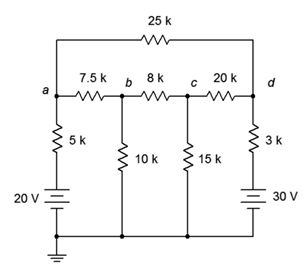Figure 7.6.8

23. Using mesh analysis, determine the value of $$V_{ad}$$ for the circuit shown in Figure 7.6.8 .

24. For the circuit shown in Figure 7.6.8 , use mesh analysis to determine the current through the 3 k$$\Omega$$ resistor.

25. Given the circuit in Figure 7.6.9 , write the mesh loop equations.Figure 7.6.9

26. Using mesh analysis, determine the value of $$V_e$$ for the circuit shown in Figure 7.6.9 .

27. For the circuit shown in Figure 7.6.9 , use mesh analysis to determine the current through the 9 k$$\Omega$$ resistor.

28. Given the circuit in Figure 7.6.10 , write the mesh loop equations and the associated determinants.Figure 7.6.10

29. Using mesh analysis, determine the value of $$V_{bc}$$ for the circuit shown in Figure 7.6.10 .

30. Given the circuit shown in Figure 7.6.10 , use mesh analysis to determine the current through the 600 $$\Omega$$ resistor.

31. Given the circuit in Figure 7.6.11 , write the mesh loop equations.Figure 7.6.11

32. Using mesh analysis, determine the value of $$V_{bc}$$ for the circuit shown in Figure 7.6.11 .

33. Given the circuit shown in Figure 7.6.11 , use mesh analysis to determine the current through the 800 $$\Omega$$ resistor.

34. For the circuit in Figure 7.6.12 , write the mesh loop equations.Figure 7.6.12

35. Using mesh analysis, determine the value of $$V_a$$ for the circuit shown in Figure 7.6.12 .

36. For the circuit shown in Figure 7.6.12 , use mesh analysis to determine the current through the 3 k$$\Omega$$ resistor.

37. Given the circuit in Figure 7.6.13 , write the mesh loop equations.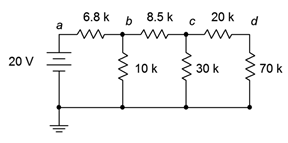Figure 7.6.13

38. Using mesh analysis, determine the value of $$V_c$$ for the circuit shown in Figure 7.6.13 .

39. For the circuit shown in Figure 7.6.13 , use mesh analysis to determine the current passing through the 8.5 k$$\Omega$$ resistor.

40. Given the circuit in Figure 7.6.14 , write the mesh loop equations (consider using source conversion).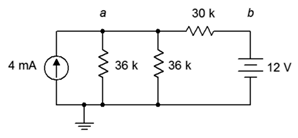Figure 7.6.14

41. Using mesh analysis, determine the value of $$V_a$$ for the circuit shown in Figure 7.6.14 .

42. For the circuit shown in Figure 7.6.14 , use mesh analysis to determine the current passing through the 30 k$$\Omega$$ resistor.

43. Given the circuit in Figure 7.6.15 , write the mesh loop equations (consider using source conversion).Figure 7.6.15

44. Using mesh analysis, determine the value of $$V_b$$ for the circuit shown in Figure 7.6.15 .

45. For the circuit shown in Figure 7.6.15 , use mesh analysis to determine the current through the 5 k$$\Omega$$ resistor.

46. Given the circuit in Figure 7.6.16 , write the node equations.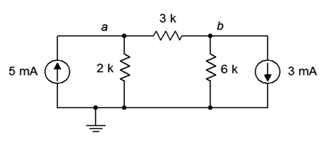Figure 7.6.16

47. Using nodal analysis, determine the value of $$V_b$$ for the circuit shown in Figure 7.6.16 .

48. For the circuit shown in Figure 7.6.16 , use nodal analysis to determine the current through the 3 k$$\Omega$$ resistor.

49. Given the circuit in Figure 7.6.17 , write the node equations.Figure 7.6.17

50. Using nodal analysis, determine the value of $$V_b$$ for the circuit shown in Figure 7.6.17 .

51. For the circuit shown in Figure 7.6.17 , use nodal analysis to determine the current passing through the 12 k$$\Omega$$ resistor.

52. Given the circuit in Figure 7.6.18 , write the node equations.Figure 7.6.18

53. Using nodal analysis, determine the value of $$V_{ba}$$ for the circuit shown in Figure 7.6.18 .

54. For the circuit shown in Figure 7.6.18 , use nodal analysis to determine the current passing through the 100 $$\Omega$$ resistor.

55. Given the circuit in Figure 7.6.19 , write the node equations.Figure 7.6.19

56. Using nodal analysis, determine the value of $$V_{ac}$$ for the circuit shown in Figure 7.6.19 .

57. For the circuit shown in Figure 7.6.19 , use nodal analysis to determine the current passing through the 20 k$$\Omega$$ resistor.

58. Given the circuit in Figure 7.6.20 , write the node equations.Figure 7.6.20

59. Using nodal analysis, determine the value of $$V_b$$ for the circuit shown in Figure 7.6.20 .

60. For the circuit shown in Figure 7.6.20 , use nodal analysis to determine the current through the 3 k$$\Omega$$ resistor.

61. Given the circuit in Figure 7.6.21 , write the node equations.Figure 7.6.21

62. Using nodal analysis, determine the value of $$V_b$$ for the circuit shown in Figure 7.6.21 .

63. For the circuit shown in Figure 7.6.21 , use nodal analysis to determine the current through the 40 $$\Omega$$ resistor.

64. Given the circuit in Figure 7.6.13 , write the node equations.

65. Using nodal analysis, determine the value of $$V_d$$ for the circuit shown in Figure 7.6.13 .

66. For the circuit shown in Figure 7.6.13 , use nodal analysis to determine the current passing through the 20 k$$\Omega$$ resistor.

67. Given the circuit in Figure 7.6.14 , write the node equations using the general approach. Do not use source conversions.

68. Using nodal analysis, determine the value of $$V_{ab}$$ for the circuit shown in Figure 7.6.14 .

69. For the circuit shown in Figure 7.6.14 , use nodal analysis to determine the current passing through the 30 k$$\Omega$$ resistor.

70. Given the circuit in Figure 7.6.15 , write the node equations.

71. Using nodal analysis, determine the value of $$V_b$$ for the circuit shown in Figure 7.6.15 .

72. For the circuit shown in Figure 7.6.15 , use nodal analysis to determine the current through the 4 k$$\Omega$$ resistor.

73. For the circuit of Figure 7.6.22 , determine $$V_b$$.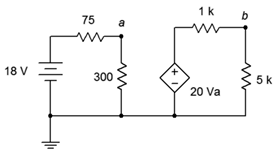Figure 7.6.22

74. For the circuit of Figure 7.6.23 , determine $$V_c$$.Figure 7.6.23

75. Given the circuit of Figure 7.6.24 , determine $$V_b$$.Figure 7.6.24

76. Find the current through the 10 k$$\Omega$$ resistor given the circuit of Figure 7.6.25 .Figure 7.6.25

77. Given the circuit of Figure 7.6.26 , determine $$V_c$$.Figure 7.6.26

78. In the circuit of Figure 7.6.27 , determine $$V_a$$.Figure 7.6.27

79. For the circuit of Figure 7.6.28 , determine $$V_a$$.Figure 7.6.28

80. For the circuit of Figure 7.6.29 , determine $$V_a$$.Figure 7.6.29

## Challenge

81. Given the circuit in Figure 7.6.8 , write the node equations.

82. Using nodal analysis, determine the value of $$V_b$$ for the circuit shown in Figure 7.6.8 .

83. For the circuit shown in Figure 7.6.8 , use nodal analysis to determine the current through the 3 k$$\Omega$$ resistor.

84. Given the circuit in Figure 7.6.6 , write the node equations.

85. Using nodal analysis, determine the value of $$V_c$$ for the circuit shown in Figure 7.6.6 .

86. For the circuit shown in Figure 7.6.6 , use nodal analysis to determine the current through the 8 k$$\Omega$$ resistor.

87. Given the circuit in Figure 7.6.10 , write the node equations and the associated determinants.

88. Using nodal analysis, determine the value of $$V_{bc}$$ for the circuit shown in Figure 7.6.10 .

89. For the circuit shown in Figure 7.6.10 , use nodal analysis to determine the current through the 2 k$$\Omega$$ resistor.

90. Given the circuit of Figure 7.6.30 , determine $$V_c$$.Figure 7.6.30

91. Find the current through the 10 k$$\Omega$$ resistor in the circuit of Figure 7.6.31 .Figure 7.6.31

92. Given the circuit of Figure 7.6.32 , determine $$V_c$$.Figure 7.6.32

93. Given the circuit of Figure 7.6.33 , determine the current through the 5 k$$\Omega$$ resistor.Figure 7.6.33

94. For the circuit of Figure 7.6.34 , determine $$V_b$$.Figure 7.6.34

95. For the circuit of Figure 7.6.35 , determine $$V_c$$.Figure 7.6.35

96. For the circuit of Figure 7.6.36 , determine $$V_a$$.Figure 7.6.36

97. For the circuit of Figure 7.6.37 , determine $$V_b$$.Figure 7.6.37

## Simulation

98. Perform a DC bias simulation on the circuit depicted in Figure 7.6.7 to verify the component currents.

99. Perform a DC bias simulation on the circuit depicted in Figure 7.6.9 to verify the component currents.

100. Perform a DC bias simulation on the circuit depicted in Figure 7.6.7 to verify the loop currents and node voltages.

101. Perform a DC bias simulation on the circuit depicted in Figure 7.6.16 to verify the node voltages.

102. Perform a DC bias simulation on the circuit depicted in Figure 7.6.19 to verify the node voltages.

103. Perform a DC bias simulation on the circuit depicted in Figure 7.6.20 to verify the node voltages.

This page titled 5.6: Exercises is shared under a CC BY-NC-SA license and was authored, remixed, and/or curated by James M. Fiore.